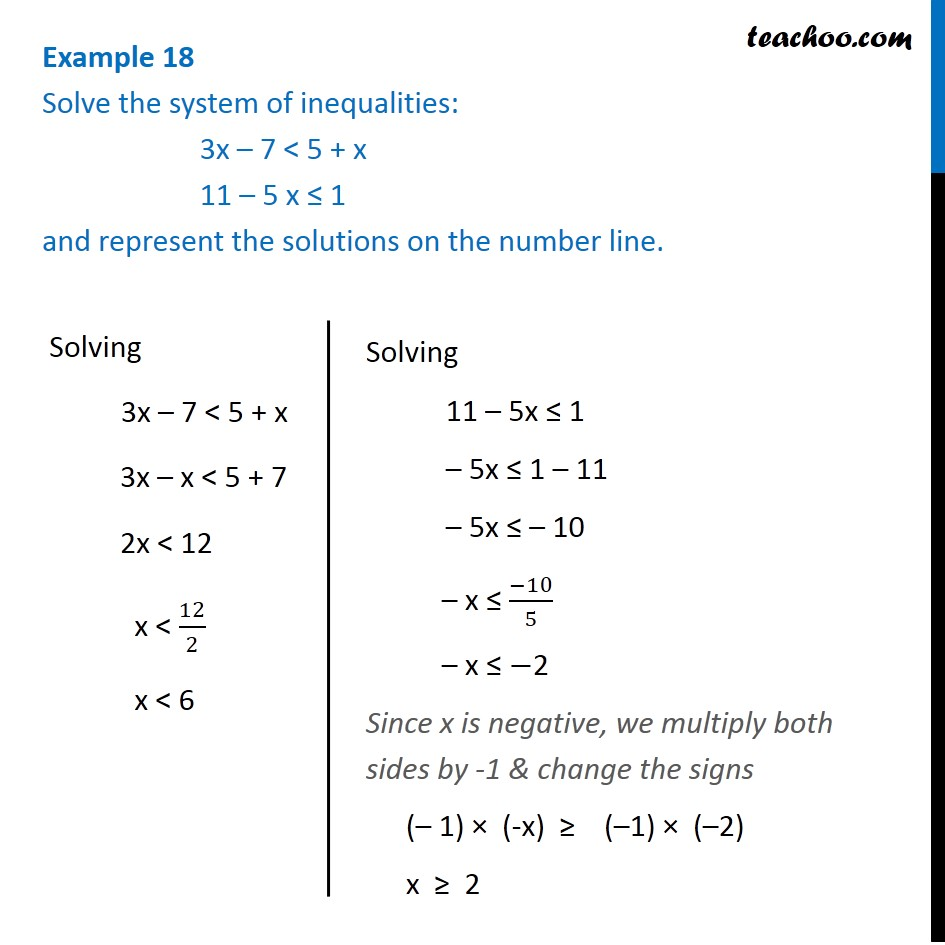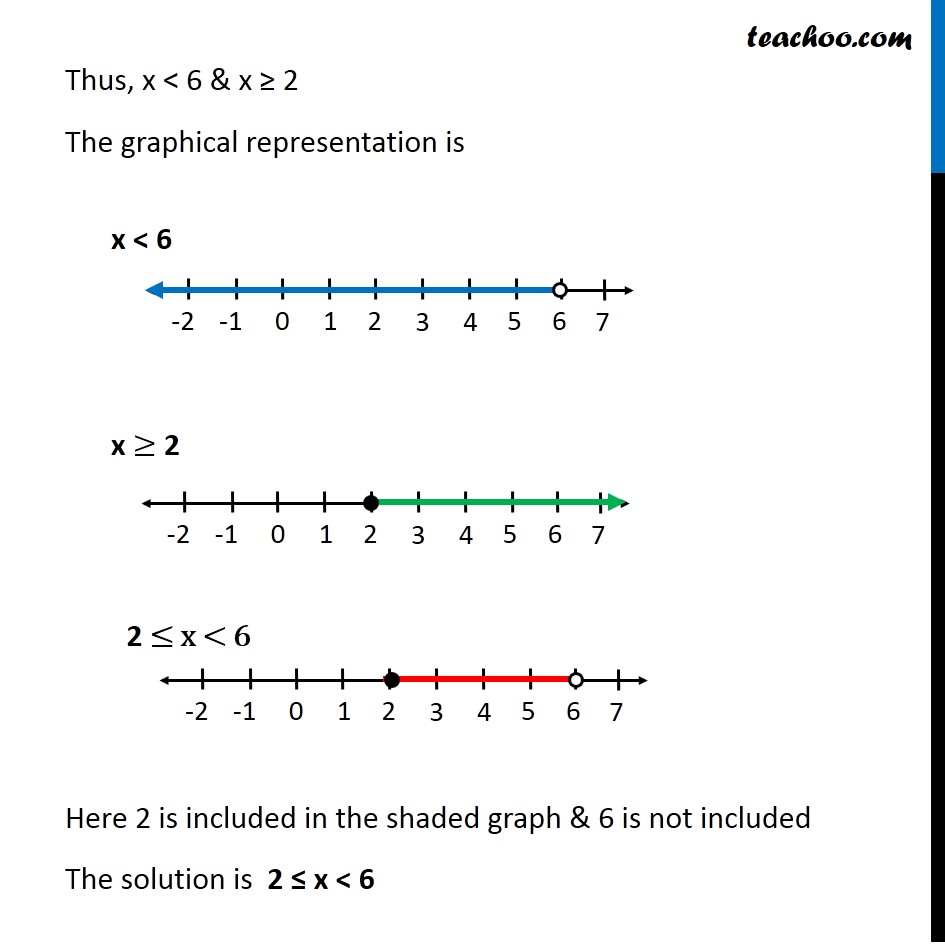Subscribe to our Youtube Channel - https://you.tube/teachoo

1. Chapter 6 Class 11 Linear Inequalities
2. Concept wise
3. Solving on number line (two graphs)

Transcript

Example 18 Solve the system of inequalities: 3x – 7 < 5 + x 11 – 5 x ≤ 1 and represent the solutions on the number line. Solving 3x – 7 < 5 + x 3x – x < 5 + 7 2x < 12 x < 12/2 x < 6 Solving 11 – 5x ≤ 1 – 5x ≤ 1 – 11 – 5x ≤ – 10 – x ≤ (−10)/5 – x ≤ −2 Since x is negative, we multiply both sides by -1 & change the signs (– 1) × (-x) ≥ (–1) × (–2) x ≥ 2 Thus, x < 6 & x ≥ 2 The graphical representation is Here 2 is included in the shaded graph & 6 is not included The solution is 2 ≤ x < 6

Solving on number line (two graphs)